Command Reference : Matrix Language Reference

 @cor
Pearson product moment correlation.
Syntax: @cor(x)
x: matrix
Return: sym
Compute the correlation between columns of a matrix.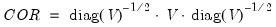where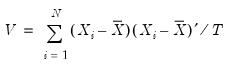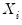is the column vector corresponding to the i-th row of the matrix,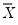are the corresponding means, and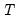is the number of rows of the matrix.
Examples
matrix x = @mnrnd(300, 5)
sym cov = @cov(@inner(x))
computes the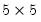non d.f. corrected covariance matrix of the randomly generated X.
Cross-references